Home » Algorithms Order Of Growth

# Algorithms Order Of Growth

The Big O notation, the theta notation and the omega notation are asymptotic notations to measure the order of growth of algorithms when the magnitude of inputs increases.

In the previous article – performance analysis – you learned that algorithm executes in steps and each step takes a “constant time“. You can count the number of steps and then arrive at total computing time required for the algorithm.

You also learned that complexity affects the performance of the algorithm. These complexities are space complexity and time complexity.

Complexity means how do use of resources usage scale for an algorithm, whereas performance account for actual resources (e.g disk, memory, CPU time) used by algorithms during execution. Complexity affects the performance, performance does not change complexity.

If you leave fixed space like variables, constants etc, and fixed time like compile time, the complexity depends on instance characteristics (operations or steps ) of the algorithm like input size or magnitude.

## What is a suitable method to analyze the algorithm?

When we consider instance characteristics which are the number of operations performed by an algorithm to solve a problem of size n.
You want to analyze three cases.

1. Worst Case Performance
2. Average Case Performance
3. Best Case Performance

Worst Case Performance: given an instance of a problem with the maximum number of operations what is the time required for the algorithm to solve the problem. This is the worst case analysis for the algorithm.

Average Case Performance: the instance of a problem has the average number of operations that the algorithm needs to solve. The time to complete such operations is the average case analysis of the algorithm.

Best Case Performance: the problem is already in a solved state or needs fewer steps to solve.

While analyzing an algorithm you should be more concerned about the worst case than average or best case. It is better to compare two algorithms based on the worst-case scenario.

### Notations for Complexity

The number of operations for an algorithm when counted will give a function.

For example

an2+ bn + c

This equation represents an exact step count for an algorithm. You do not need an exact count for the algorithm because when we analyze the algorithm for the worst case, consider only higher-order term and drop the lower order terms because they are insignificant when the input is huge.

therefore,

an2 + bn + c

becomes

O(n2)

This symbol is known as Landau symbol or Big O notation named after German mathematician Edmund Landau. It tells us the fastest growing term in the function called the Order or rate of growth. That is why the lower order terms become insignificant and dropped.

The asymptotic notations such as Big O is used to describe the running time of the algorithms. There are other notations to describe the running time as well.

Suppose T(n) is the function of the algorithm for input size n,

then running time is given as

T(n) = O(n2)

### Formal Definition of Big O

Let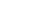and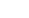be two functions, then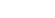is asymptotic upper bound tofor a problem size of n.

For a given functionthe functionis a set of functions

O(g(n))= {f(n): there exist positive contants c and n0 such that 0≤f(n) ≤cg(n) for all n ≥n0

The graph of the function is given by the following.

### Formal Definition of Theta-Notation

Letandbe two functions. Then the function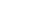gives asymptotic upper bound and asymptotic lower bound, its means that the functionis “sandwiched” between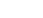and.

θ(g(n) = {f(n): there exist positive constants c1,c2 and n0 such that 0 ≤c1g(n) ≤ f(n) ≤c2g(n) for all n ≥ n0

The graph of Theta notation is given below.

### Formal Definition of Omega-Notation

Letandbe two functions. Then the function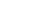gives  asymptotic lower bound, its means that the functionis greater than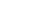.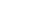= { f(n) : there exists positive constantsand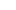such that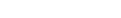for all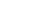The graph of Omega notation is given below.